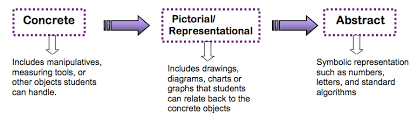# Mathematics

Mathematics in Langford Village Academy is taught using the Mastery approach which emphasises a mastery curriculum that involves whole-class teaching, with all pupils being taught the same concepts at the same time. Our mathematics curriculum aims to enable children to become:

Fluent in the fundamentals of mathematics, including through varied and frequent practice with increasingly complex problems over time, so that pupils develop conceptual understanding and the ability to recall and apply knowledge rapidly and accurately

Reason mathematically by following a line of enquiry, conjecturing relationships and generalisations, and developing an argument, justification or proof using mathematical language

Can solve problems by applying their mathematics to a variety of routine and non-routine problems with increasing sophistication, including breaking down problems into a series of simpler steps and persevering in seeking solutions.

To achieve these aims we employ concrete and pictorial representations alongside the abstract to develop and reinforce understanding for all children, regardless of ability and attainment.Our Mathematics Curriculum is planned using the White Rose Maths (2017) Teachers use this resource to plan teaching sequences to suit the needs of the children in their class. Although number is at the heart of the Mastery curriculum, mathematics at Langford also includes Measurement (length, height, weight, volume, time, money, temperature), Geometry (shape, position and direction) and Statistics.

### Time tables

Multiplication tables are important building blocks in the children’s learning of Maths. Secure and quick recall of times tables facts allows children to confidently tackle problems requiring multiplication and division as they progress through the school. Multiplication skills and facts are important outside of school, at home and in the workplace.

Year Group Multiplication and Division Facts
1

Count in multiples of 2,5 and 10

Solve one-step problems involving multiplication by calculating the answer using concrete objects, pictorial representations and arrays.

2

Recall and use multiplication and division facts for 2, 5 and 10 multiplication tables, including recognising odd and even numbers.

3

Recall and use multiplication and division facts for the 3,4 and 8 multiplication tables

4

Recall and use multiplication and division facts for multiplication tables up to 12 x 12

5

Recall and use multiplication and division facts for multiplication tables up to 12 x 12

Identify multiples and factors

6

Recall and use multiplication and division facts for multiplication tables up to 12 x 12

Identify common factors, common multiples and prime numbers